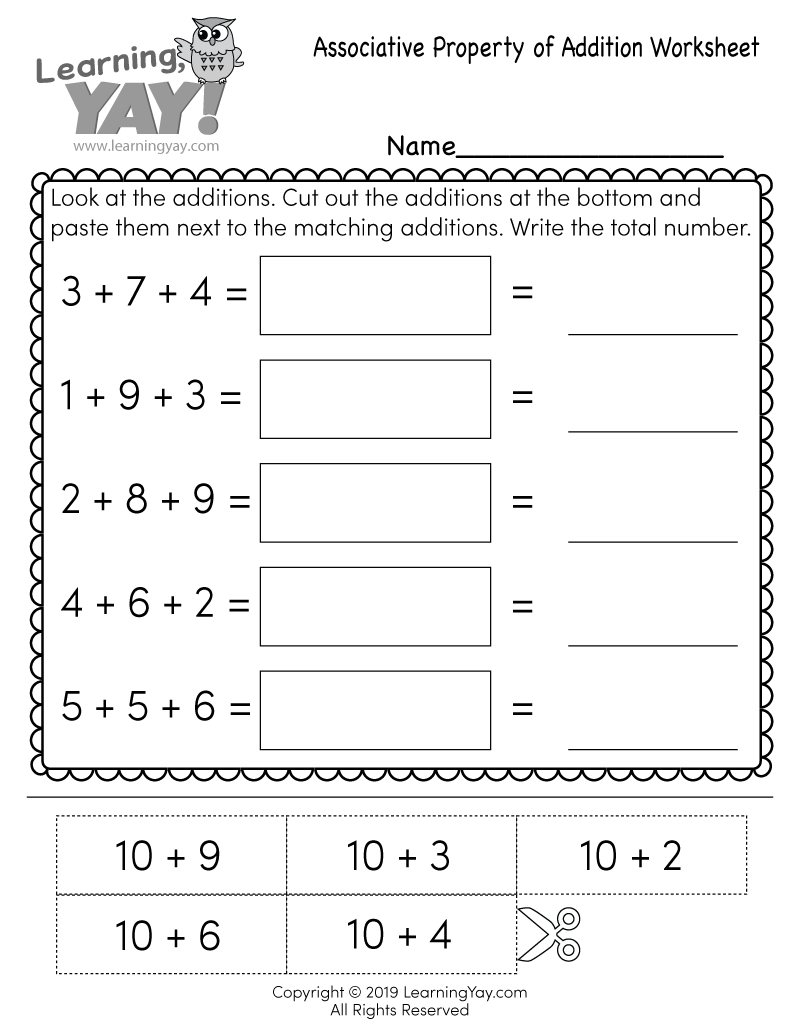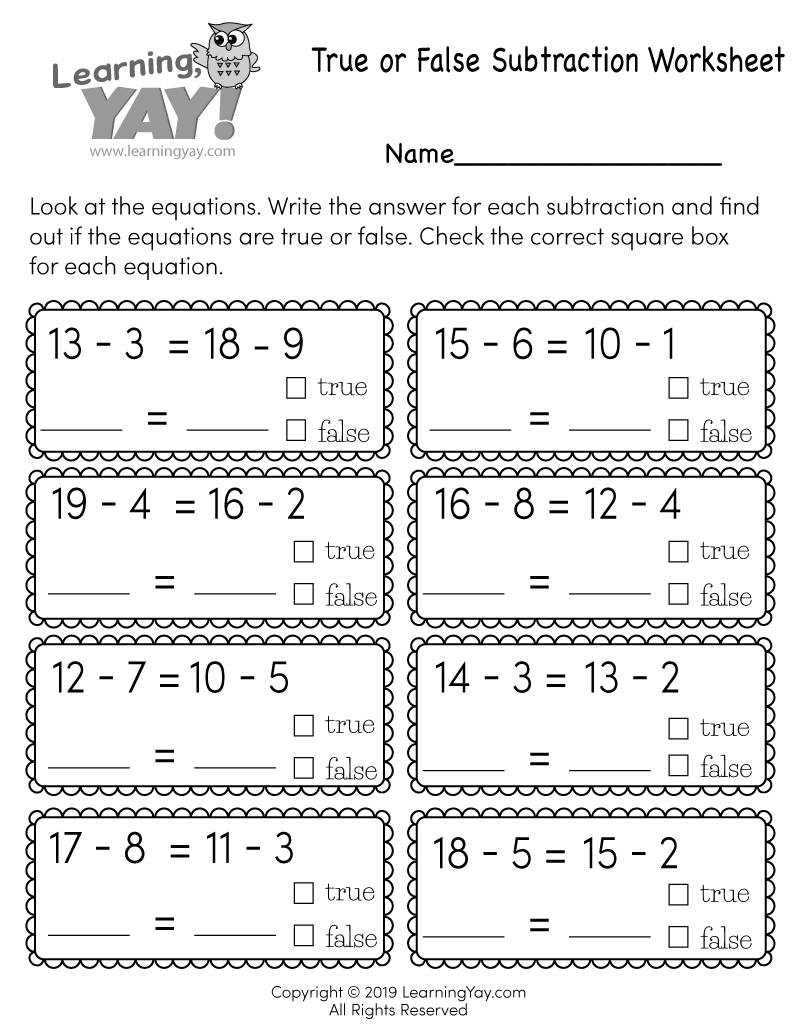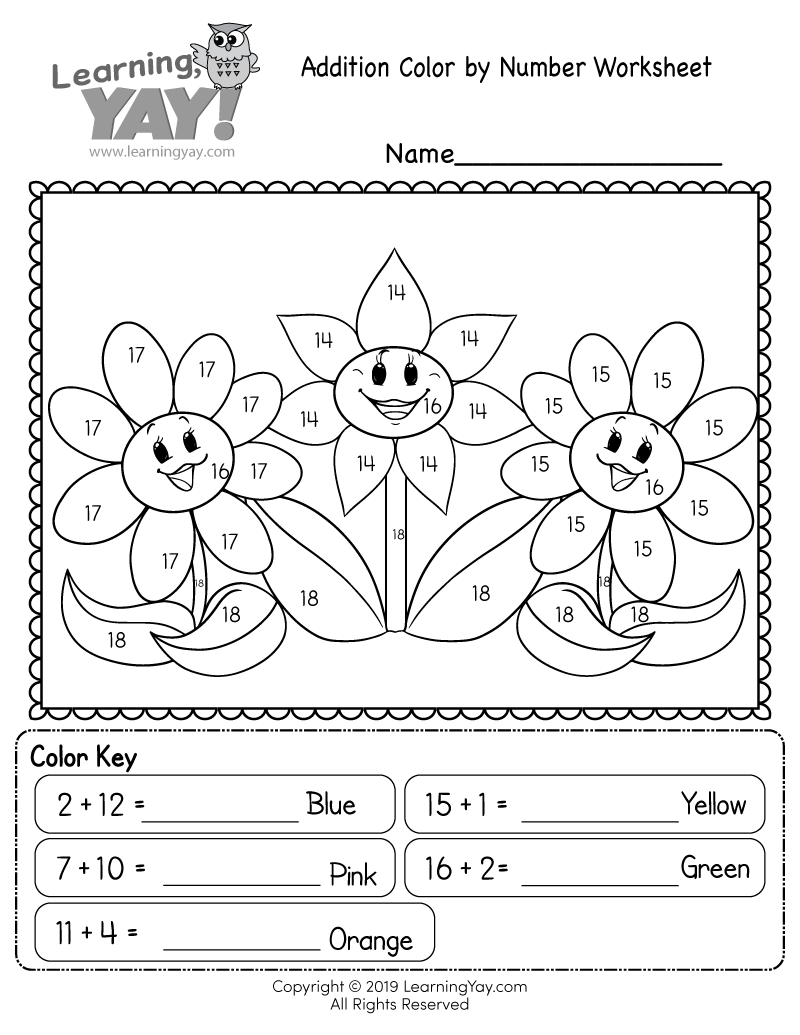# Associative Property of Addition Worksheet

Students can practice mental math while completing a simple cut and paste activity that will help them learn the associative property of addition. We want students to grasp the concept that answers to addition problems will remain the same when you regroup numbers.This free printable math worksheet is aligned to first grade Common Core standards. It is an excellent resource for teachers and parents who are teaching the following standard.
##### 1.OA.B.3 - Common Core ID

Apply properties of operations as strategies to add and subtract. Examples: If 8 + 3 = 11 is known, then 3 + 8 = 11 is also known. (Commutative property of addition.) To add 2 + 6 + 4, the second two numbers can be added to make a ten, so 2 + 6 + 4 = 2 + 10 = 12. (Associative property of addition.)

Common Core » 1st Grade Math Standards » Operations & Algebraic Thinking » Understand and apply properties of operations and the relationship between addition and subtraction. » 1.OA.B.3

## There are multiple ways to get this addition worksheet.

### You might also like these math worksheets: